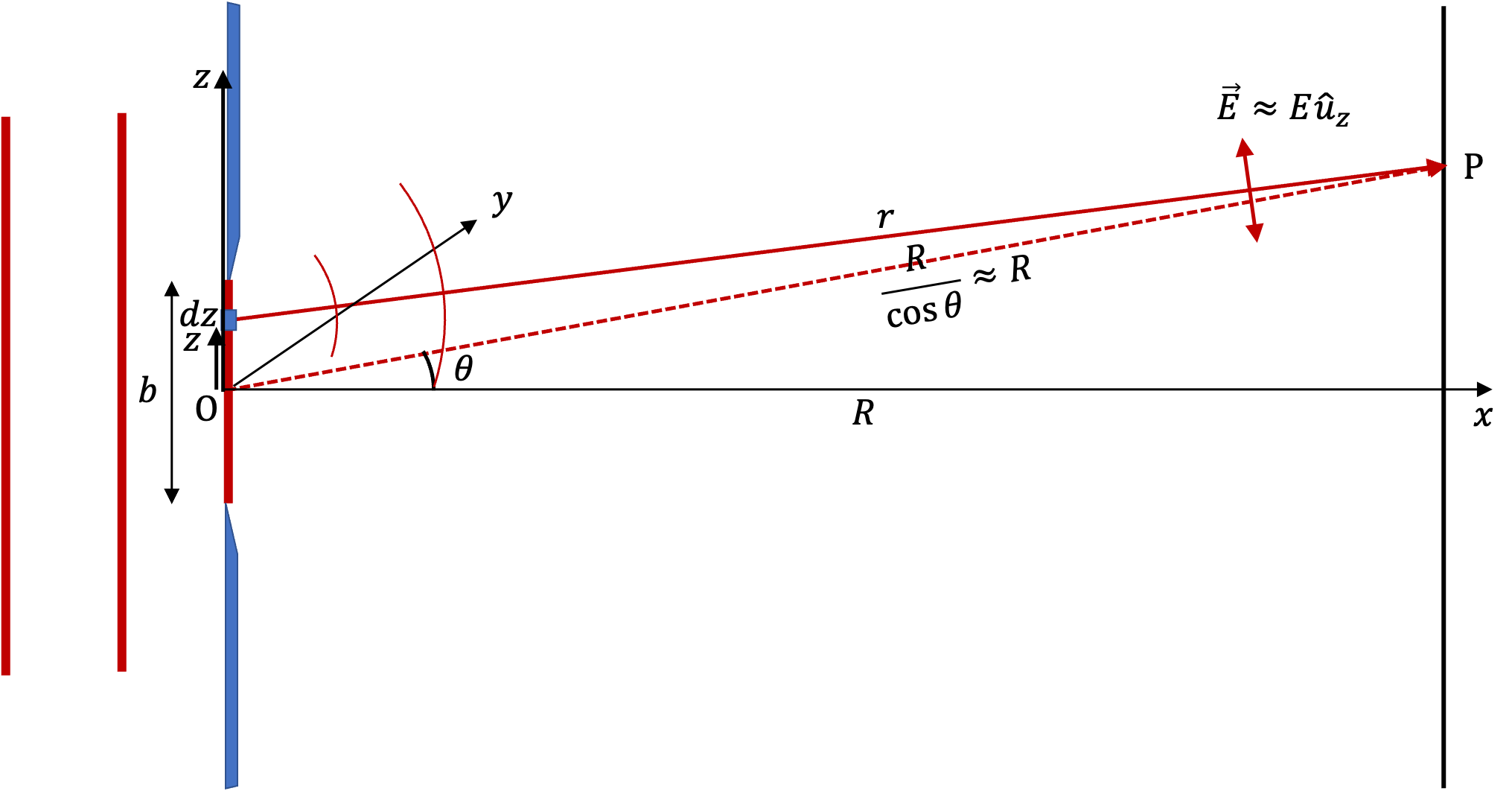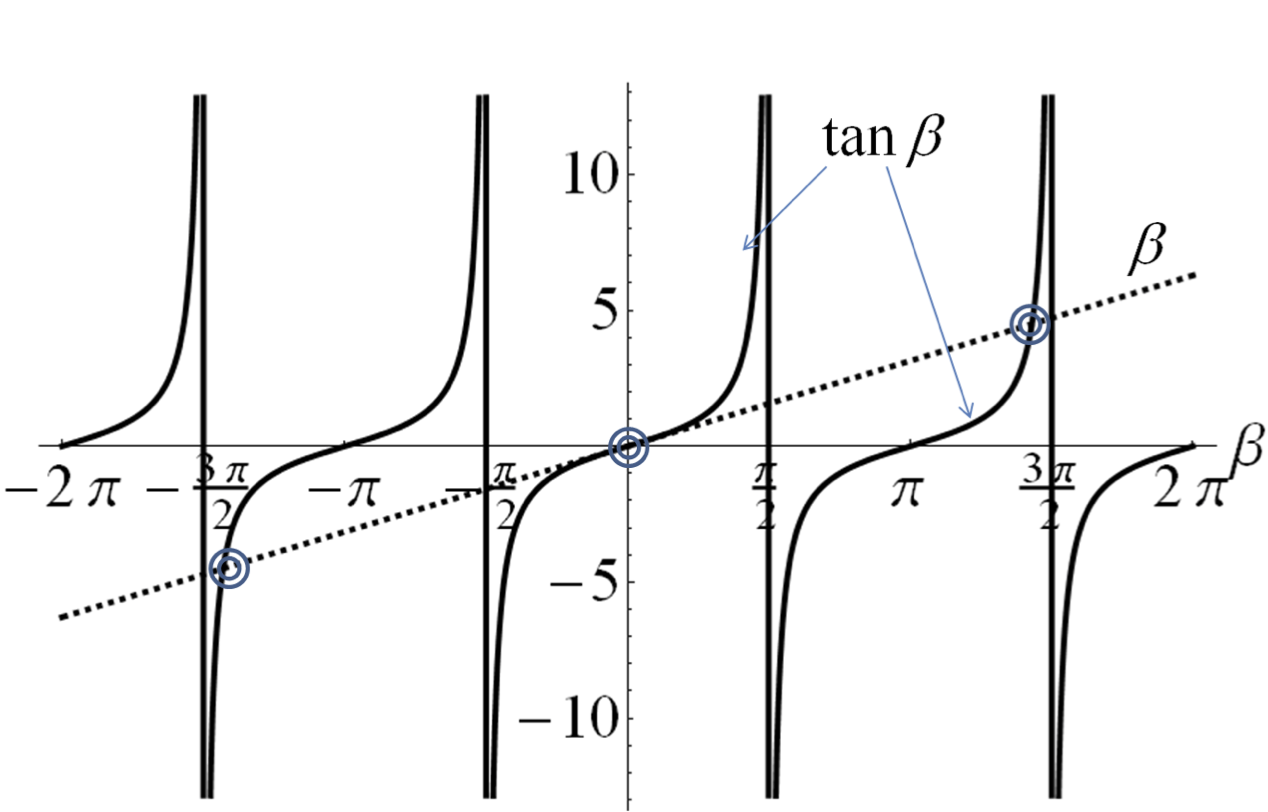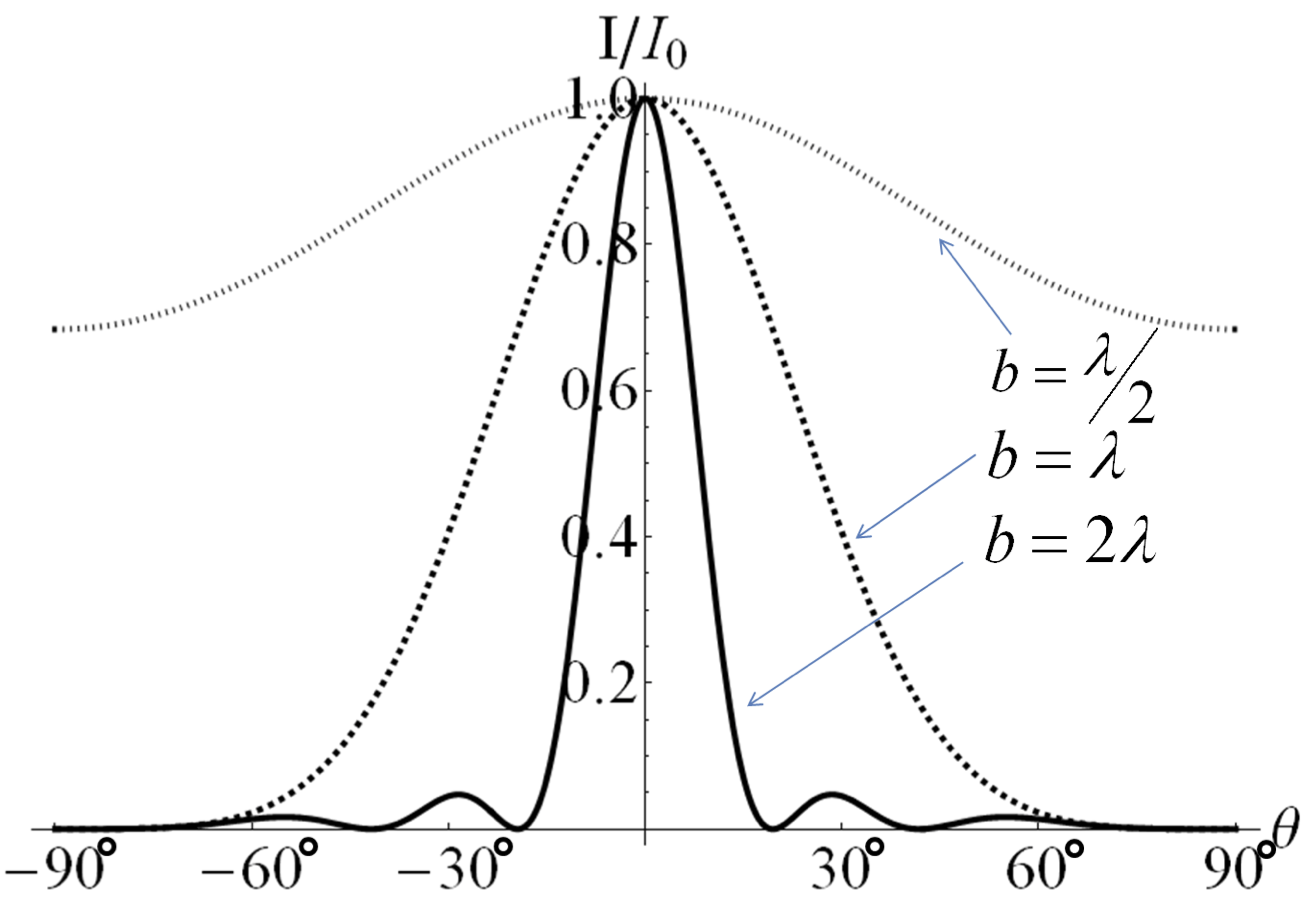## Section51.4Intensity in Diffraction Pattern

###### (Calculus) Formula for Intenssity.

It turns out that figuring out intensity in the diffraction pattern is not so easy as finding the directions of the minima. We must apply the superposition principle to the waves, and then, find the time-average of the square of the sum.

We will use Figure 51.4.1 to perform out calculations. I have placed a $z$ axis at the slit to refer to parts of the wavefront there. Let $y$ axis be pointed into the page and $x$ axis pointed to the right. Note that this figure shows only a cross-section of the wavefront at the slit since the wavefront is parallel to the $yz$ plane.Figure 51.4.1. Geometry for the calculation of Fraunhofer diffraction from plane wave at a slit. We assume electric field to be mostly along $z$ axis. Here $\hat u_z$ is unit vector towards positive $z$ axis. $dz$ shows a small part of the wavefront at coordinate $z\text{.}$

We will also assume that electric field is mostly the $z$ component. Therefore, work with just the $E_z$ wave and call $E_z$ by $E\text{.}$ Let $A$ denote the voltage per unit length at the wavefront. Then, we can write the electric field wave at point P on the screen which started at $dz$ of the wavefront as a spherical wave as follows.

\begin{equation} dE = \frac{A dz}{r}\cos(kr - \omega t),\label{eq-electric-field-dE-from-dz-as-spherical-wave-original}\tag{51.4.1} \end{equation}

$Adz/r$ is the amplitude of this wave when it arrives at P, $k$ is the wavenumber, which is related to wavelength $\lambda$ by

\begin{equation*} k = \frac{2\pi}{\lambda}, \end{equation*}

$\omega$ is the angular frequency, which is related to the frequency $f$ by

\begin{equation*} \omega = 2\pi f. \end{equation*}

Simplifying $r\text{:}$

From triangle, whose vertices are O, P, and $dz\text{,}$ we get the following for $r^2\text{.}$

\begin{align*} r^2 \amp = \left( \frac{R}{\cos\theta} \right)^2 + z^2 - 2 \frac{zR}{\cos\theta}\cos\left( \frac{\pi}{2} - \theta\right) \\ \amp = \left( \frac{R}{\cos\theta} \right)^2 + z^2 - 2 \frac{zR}{\cos\theta}\sin \theta. \end{align*}

Now, since $z$ will be a point on the wavefront at the slit, its range of value is limited,

\begin{equation*} -\frac{b}{2} \le z \le \frac{b}{2}. \end{equation*}

Since $\frac{R}{\cos\theta} \gt \gt b\text{,}$ we can drop $z^2$ in $r^2$ to get

\begin{equation*} r^2 \approx \left( \frac{R}{\cos\theta} \right)^2 - 2 \frac{zR}{\cos\theta}\sin \theta. \end{equation*}

Taking square root of both sides we get

\begin{equation*} r = \frac{R}{\cos\theta} \left( 1 - 2 \frac{z \cos\theta}{R}\sin \theta \right)^{1/2}. \end{equation*}

Here since $|z|\lt \lt R\text{,}$ we can expand the quantity in parenthesis and keep upto two terms so that we have dependence on $z$ still there.

\begin{equation*} r = \frac{R}{\cos\theta} - z \sin\theta. \end{equation*}

We make one more simplification here. Since $\theta$ will be small, we can replace $\cos\theta$ by $1\text{.}$ To be consistent, we could replace $\sin\theta$ by $\theta$ in radians, but, it is customary to leave $\sin\theta$ alone here. Finally, we have the following simplified expression for $r\text{.}$

\begin{equation} r = R - z \sin\theta.\label{eq-simplified-r-single-slit}\tag{51.4.2} \end{equation}

Electric Field at P:

In Eq. (51.4.1), distance $r$ appears in the amplitude $\frac{Adz}{r}$ and in phase $kr - \omega t\text{.}$ Since $|z\sin \theta| \lt\lt R$ we may be tempted to drop $z\sin \theta$ altogether. Since $kr$ in the phase has a large $k\text{,}$ small changes in $kr$ have large impact on sine of $kr$ . Therefore, in the phase we must keep $z\sin \theta\text{.}$ Dropping $z\sin \theta$ in the the amplitude $\frac{Adz}{r}$ does not have much impact. Thus, Eq. (51.4.1) simplifies to

\begin{equation*} dE \approx \frac{(Adz)}{R}\sin\left[ k(R-z\sin\theta) - \omega t\right] \end{equation*}

Now, we just integrate this over the wavefront, from $z=-b/2$ to z=+b/2, to get the electric field at P which is in the direction $\theta$ from the slit.

\begin{equation} E_P = \frac{A}{R} \left[ \frac{2\sin\left(\frac{kb}{2}\sin\theta\right)}{kb\sin\theta} \right]\ \sin\left[ kR - \omega t\right].\label{eq-electric-field-at-screen-single-slit-diffraction}\tag{51.4.3} \end{equation}

Inensity in direction $\theta\text{:}$

Intensity at in the direction $\theta$ is proportional to time average of this fluctuating field. Since time averaging of $\sin^2(\omega t)$ and $\cos^2(\omega t)$ give $\frac{1}{2}\text{,}$ after calculations, we end up with the following for intensity.

\begin{equation} I(\theta)=\epsilon_0 c \left( \frac{bA}{R} \right)^2 \left[ \frac{\sin\beta}{\beta} \right]^2,\label{eq-electric-intensity-in-direction-theta-single-slit-diffraction}\tag{51.4.4} \end{equation}

where symbol $\beta$ stands for

\begin{equation} \beta = \frac{\pi b \sin\theta}{\lambda}.\label{eq-beta-in-terms-of-theta-single-slit}\tag{51.4.5} \end{equation}

Let us denote the intensity in the $\theta_0$ direction by $I_0\text{.}$ Since $sin x/x = 1$ when $x=0\text{,}$ from Eq. (51.4.4) we get

\begin{equation} I_0 = \epsilon_0 c \left( \frac{bA}{R} \right)^2.\tag{51.4.6} \end{equation}

This helps us write intensity in direction $\theta$ in terms of $I_0\text{.}$ We rewrite Eq. (51.4.4) as

\begin{equation} I(\theta) = I_0 \left( \frac{\sin\beta(\theta)}{\beta(\theta)} \right)^2.\label{eq-electric-intensity-in-direction-theta-single-slit-final}\tag{51.4.7} \end{equation}

### Subsection51.4.1Diffraction Maxima and Minima

We now use the formula for intensity (51.4.7) to find the values of $\beta$ in which intensity will have maxima and minima. After we get these $\beta$ values, we use Eq. (51.4.5) to obtain angles $\theta$ which give us the directions from the center of the slit.

Diffraction Minima

First we notice that when \beta = 0, $sin\beta /\beta$ is not zero, but it is equal to 1. For $\beta = m^\prime \pi$ for non-zero integer $m\text{,}$ $\sin\beta /\beta$ is zero. Therefore, we have all the betas for which we will have zero intensity, which are the minima here. Let us give subscripts to these betas.

\begin{equation} \text{Minima: }\ \beta_{m^\prime} = m^\prime \pi\ \ (m^\prime \text{ nonzero integer})\tag{51.4.8} \end{equation}

Using Eq. (51.4.5), these will corresponds to the following in terms of angles. Giving subscripts to these thetas we have.

\begin{equation} \frac{\pi b \sin\theta_{m^\prime}}{\lambda} = m^\prime \pi.\tag{51.4.9} \end{equation}

Rearranging this gives us the result we obtained by a trick in Subsection 51.3.1.

\begin{equation} \text{Minima: }\ b\sin\theta = m^\prime \lambda \ \ (m^\prime \text{ nonzero integer})\tag{51.4.10} \end{equation}

Diffraction Maxima

The intensity maxima do not occur at points halfway between the minima. Instead we must find $\beta$ values at the maxima of intensity $I$ by treating $I(\beta)$ as a function of $\beta\text{.}$ By setting the derivative $dI/d\beta$ to zero, we will get both maxima and minima, and since we already know the minima, we will identify the maxima by removing the minima from the list. $dB/d\beta=0$ gives

\begin{equation*} \frac{1}{\beta^3}\sin(\beta) \left(\beta\cos\beta -\sin\beta \right) = 0. \end{equation*}

Of the three factors, we can drop $\/\beta^3=0\text{,}$ and ge the following conditions for maxima and minima.

\begin{align} \amp \sin\beta = 0.\label{eq-extrema-of-intensity-sin-beta-equal-zero}\tag{51.4.11}\\ \amp \beta\cos\beta -\sin\beta = 0.\label{eq-extrema-of-intensity-tan-beta-equal-beta}\tag{51.4.12} \end{align}

The first condition includes one maximum at $\beta = 0\text{,}$ and the rest of the betas are minima as discussed above.

The second equation corresponds to only maxima. You can verify that this is the case by using second derivative test. Now, you shoudl notice that, unlike solving $\sin\beta = 0\text{,}$ solving $\beta\cos\beta -\sin\beta = 0$ is no easy task. This equation is a transcendental equation and can be solved graphically.

Before, we solve Eq. (51.4.12), by dividing by $\cos\theta$ we convert this equation to the following since it is easier to see when $\tan\beta$ and $\beta$ cross than when $beta\cos\beta -\sin\beta = 0$ and $\sin\beta$ cross.

\begin{equation*} \tan\beta = \beta. \end{equation*}

We now plot $\tan \beta$ and $\beta$ versus $\beta$ as shown in Figure 51.4.2. The approximate solutions for the maxima can be read off from the graphs, for instance, 0, $\pm\frac{3}{2}\pi\text{,}$ $\pm\frac{5}{2}\pi\text{,}$ etc.Figure 51.4.2. The graphical solution of $\tan\beta = \beta.\text{.}$

A more precise and expanded graph gives solutions to be

\begin{align*} \text{Maxima: } \amp \\ \beta_0 \amp = 0, \\ \beta_1 \amp = 1.4303\pi \approx \frac{3}{2}\pi,\ \ \beta_{-1} = -1.4303\pi \approx -\frac{3}{2}\pi,\\ \beta_2 \amp = 2.4590\pi \approx \frac{5}{2}\pi,\ \ \beta_{-2} = -2.4590\pi \approx -\frac{5}{2}\pi,\\ \beta_3 \amp = 3.4707\pi \approx \frac{7}{2}\pi,\ \ \beta_{-3} = -3.4707\pi \approx -\frac{7}{2}\pi,\text{ etc.} \end{align*}

The corresponding direction angles from the center of the slit will be

\begin{align} \theta_m \amp = \sin^{-1}\left(\frac{\beta_m}{\pi}\: \frac{\lambda}{b}\right)\tag{51.4.13}\\ \amp \approx \begin{cases} 0 \amp \text{ for } m = 0,\\ \pm \frac{2 m + 1}{2} \frac{\lambda}{b} \amp \text{ for } m = 1, 2, 3, \cdots. \end{cases}\tag{51.4.14} \end{align}

Intensity Plot

We can plot $I/I_0$ versus $\beta$ or versus $\theta\text{.}$ When you plot $I/I_0$ versus $\beta\text{,}$ the minima will occur at non-zero integer multiples of $\pi\text{,}$ which correspond to $\theta$ between $-\pi/2$ and $\pi/2\text{.}$

Figure 51.4.3 shows three plots of $I/I_0$ correponding to three cases of slit width compared to wavelength: $b=\lambda/2\text{,}$ $b=\lambda\text{,}$ and $b=2\lambda\text{.}$ The $b=\lambda/2$ is too narrow a slit to have diffraction minima and shows what to expect if you have very narrow opening. The $b=2\lambda$ shows five diffraction maxima, and $b=\lambda$ is the borderline case which just shows noticeably pointed spread in intensity and no minima.Figure 51.4.3. A plot of diffraction pattern for various slit widths. Only with slit width greater than the wavelength that we would observe diffraction maxima and minima.

It is worth noting that the intensity of the central peak is about 20 times the intensity of the first side peaks. Evaluating $I/I_0$ at the peaks, we find

\begin{equation*} I = \begin{cases} I_0 \amp \text{central max}\\ \frac{4}{9\pi^2} I_0\approx 0.045 I_0 \amp \text{next max}\\ \frac{4}{25\pi^2} I_0\approx 0.016 I_0 \amp \text{next max} \end{cases} \end{equation*}

If you plotted $I/I_0$ versus $\beta\text{,}$ the central peak will go from $\beta=-\pi$ to $\beta=\pi$ at the base. That is the central peak covers a range of $\Delta\beta=2\pi\text{.}$ The other peaks cover half as wide a range. For instance, the first side peak goes from $\beta=\pi$ to $\beta=2\pi\text{,}$ which gives it a range of $\Delta\beta=\pi$ only.

These ranges in $\beta$ values can be converted to corresponding ranges in $\theta$ values. For the central peak, we will get the $\theta$ ranage to be

\begin{align*} \amp \text{Central: }\ \theta \text{ from } -\sin^{-1}{\lambda/b} \text{ to } \sin^{-1}{\lambda/b},\\ \amp \text{Next peak on right: }\ \theta \text{ from } \sin^{-1}{\lambda/b} \text{ to } \sin^{-1}{2\lambda/b},\text{ etc.} \end{align*}

For small angles, these become

\begin{align*} \amp \text{Central: }\ \theta \text{ from } - \lambda/b \text{ to } \lambda/b \Longrightarrow \Delta\theta=2\lambda/b,\\ \amp \text{Next peak on right: }\ \theta \text{ from } \lambda/b \text{ to } 2\lambda/b \Longrightarrow \Delta\theta=\lambda/b,\text{ etc.} \end{align*}

Thus, in small angle case, we do see that the base of central peak is twice as wide as for other peaks. Although, width of a peak is defined at the half height, not at the base, we still get this two-to-one ratio between the width of the central maximum versus the widths of other peaks as far as width in $\beta$ is concerned.

Monochromatic light of wavelength 530 nm passes through a horizontal single slit of width 1.5 $\mu$m in an opaque plate. A screen of dimensions 2 m $\times$ 2 m is 1.2 m away from the slit. (a) Which way is the diffraction pattern spread out on the screen? (b) What are the directions of the minima? (c) What are the directions of the maxima? (d) How wide is the central bright fringe on the screen? (e) How wide is the next bright fringe on the screen?

Hint

Use diffraction conditions.

(a) Vertically, (b) $\pm 21^{\circ}\text{,}$ $\pm 45^{\circ}\text{,}$ (c) 0, $\pm 30^{\circ}\text{,}$ $\pm 60^{\circ}\text{.}$

Solution 1 (a)

(a) Since the slit is horizontal, the diffraction pattern will be vertical.

Solution 2 (b)

(b) The diffraction minima from a single slit occur when $\beta = m \pi$ for $m = \pm1,\: \pm 2,\: \cdots\text{.}$ In terms of the slit width $b$ and the direction $\theta$ vertically from the slit this condition becomes

\begin{equation*} \dfrac{\pi b \sin\:\theta_m}{\lambda} = m \pi,\ \ \Longrightarrow\ \ b\:\sin\:\theta_m = m\:\lambda,\ \ m = \pm1,\: \pm 2,\: \cdots. \end{equation*}

Using this formula we obtain the following values for $\theta_1\text{,}$ $\theta_2$ and $\theta_3\text{.}$

\begin{align*} \amp \theta_1 = \sin^{-1}\left(\dfrac{\lambda}{b} \right) = \sin^{-1}\left(\dfrac{530\:\text{nm}}{1500\:\text{nm}} \right) = 0.36\:\text{rad} = 21^{\circ},\\ \amp \theta_2 = \sin^{-1}\left(\dfrac{2\lambda}{b} \right) = \sin^{-1}\left(\dfrac{2\times530\:\text{nm}}{1500\:\text{nm}} \right) = 0.78\:\text{rad} = 45^{\circ},\\ \amp \theta_3 = \sin^{-1}\left(\dfrac{3\lambda}{b} \right) = \sin^{-1}\left(\dfrac{3\times530\:\text{nm}}{1500\:\text{nm}} \right) = \sin^{-1}\left(>1\right). \end{align*}

That means $\theta_3$ does not exist. Therefore, the positions of the first and second order spots on the screen will be given by the $y$-coordinates,

\begin{align*} \amp y_{\pm 1} =L\:\tan(\theta_{\pm1}) =(1.2\:\text{m})\:\tan(\pm 21^{\circ}) = \pm 46\:\text{cm},\\ \amp y_{\pm 2} =L\:\tan(\theta_{\pm2}) =(1.2\:\text{m})\:\tan(\pm 45^{\circ}) = \pm 1.2\:\text{cm}. \end{align*}
Solution 3 (c)

(c) Maxima when $\beta = 0, \pm 1.4303\:\pi, \pm 2.4590\:\pi, \cdots\text{.}$ Therefore, the bright spots will be in the following directions.

\begin{align*} \amp \theta_0 = 0^{\circ},\ \ \text{central bright spot},\\ \amp \theta_1 = \sin^{-1}\left( \dfrac{1.4303\:\lambda}{b}\right) = 30^{\circ},\\ \amp \theta_2 = \sin^{-1}\left( \dfrac{2.4590\:\lambda}{b}\right) = 60^{\circ}. \end{align*}

The $y$-coordinates of these bright spots are

\begin{equation*} y_0 = 0,\ \ y_{\pm 1} = 69.3\:\text{cm},\ \ y_{\pm 2} = 2.1\:\text{m}. \end{equation*}

Since the screen is only 2 m $x$ 2 m, the second order spot will not be on the screen. \item The width of the central bright spot will be between the $y_{-1}$ and $y_{+1}$ of the diffraction minima worked out in part (a).

\begin{equation*} \Delta y_{\text{central}} = y_{+1} - y_{-1} = 46\:\text{cm} - (-46\:\text{cm}) = 92\:\text{cm}. \end{equation*}
Solution 4 (d)

The $m=1$ bright spot will be between $y_{+1}$ and $y_{+2}$ of the diffraction minima. Therefore, the width of the $m=1$ bright spot will be

\begin{equation*} \Delta y_{\text{m=1}} = \left[y_{+2} - y_{+1}\right]_{\text{min}} = 1.2\:\text{m} - (46\:\text{cm}) = 74\:\text{cm}. \end{equation*}

A monochromatic light of wavelength 632.8 nm incident on a single slit has central bright spot of width 5 cm at a screen 1.6 m away. Find the width of the slit.

Hint

you can get width of the central maximum from diffraction minima around the central maximum.

40.5 $\mu$m.

Solution

From the given data we can find the direction to the $m=1$ minimum.

\begin{equation*} \theta_1 = \tan^{-1} \left( \dfrac{2.5\:\text{cm}}{160\:\text{cm}} \right) = 0.895^{\circ}. \end{equation*}

Therefore,

\begin{equation*} b\:\sin\:\theta_1 = 1\:\lambda,\ \ \Longrightarrow\ \ b = \dfrac{\lambda}{\sin\:\theta_1}. \end{equation*}

Hence,

\begin{equation*} b = \dfrac{632.8\:\text{nm}}{\sin\:0.895^{\circ}} = 40.5\:\mu\text{m}. \end{equation*}

A source of light having two wavelength 550 nm and 600 nm of equal intensity is incident on a slit of width 1.8 $\mu$m. Find the separation of the $m$ = 1 bright spots of the two wavelengths on a screen 30 cm away.

Hint

First find the directions of $m=1$ maxima for each wavelength.

2.1 cm.

Solution

From the condition for the diffraction maxima we have $b\:\sin\:\theta = 1.4303\:\lambda$ for $m=1$ maximum. Therefore, we would get following angles for the two wavelengths.

\begin{align*} \amp \theta_{550} = \sin^{-1}\left(\dfrac{1.4303\times 550\:\text{nm}}{1800\:\text{nm}} \right) = 26^{\circ},\\ \amp \theta_{600} = \sin^{-1}\left(\dfrac{1.4303\times 600\:\text{nm}}{1800\:\text{nm}} \right) = 29^{\circ}. \end{align*}

Since the distance to the screen in 30 cm the $y$-coordinates of the spots will be

\begin{align*} \amp y_{550} = 30\:\text{cm}\:\tan\:26^{\circ} = 14.6\:\text{cm},\\ \amp y_{600} = 30\:\text{cm}\:\tan\:29^{\circ} = 16.7\:\text{cm}. \end{align*}

Therefore the peaks of the spots are separated by $\Delta y = 2.1$ cm.

A single slit of width 3.0 $\mu$m is illuminated by a sodium yellow light of wavelength 589 nm. Find the intensity at a 15$^{\circ}$ angle to the axis in terms of the intensity of the central maximum which can be taken to be 1 unit.

Hint

Use the intensity formula.

$0.04$

Solution

The intensity in the diffraction pattern of a single slit is given by

\begin{equation*} I(\theta) = I(0)\:\left( \dfrac{\sin\:\beta(\theta)}{\beta(\theta)}\right)^2, \end{equation*}

where $\beta(\theta) = \pi\:b\:\sin\:\theta/\lambda\text{.}$ Let us find $\beta$ for the given angle.

\begin{equation*} \beta(15^{\circ}) = \dfrac{\pi \times 3.0\: \mu\text{m}\:\sin\:15^{\circ}}{0.589\:\mu\text{m}}=4.14\:\text{rad}. \end{equation*}